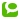I do a lot of Excel reporting using Notes data, lately.
This is pretty easy stuff, because you just have to create an Excel object and put a value into a cell.
Look at the following straightforward LotusScript code, which, put into an agent, loops over the current selected documents, and puts the form-name, creation, and modification date into an Excel spreadsheet:

Sub Initialize
Dim session As New notessession
Dim db As NotesDatabase
Dim dc As NotesDocumentCollection
Dim doc As NotesDocument
Dim i As Integer
Dim ObjExcel As Variant 'Excel Object

Set db=session.CurrentDatabase
Set dc=db.UnprocessedDocuments
If dc.Count=0 Then Exit Sub 'no documents selected in the view

Set ObjExcel = CreateObject("Excel.Application")
ObjExcel.Visible = True 'make sure the Excel sheet is visible
Call ObjExcel.Workbooks.add 'to create new worksheet

Set doc=dc.GetFirstDocument
i=1
While Not (doc Is Nothing)
ObjExcel.Cells(i,1).value=doc.form(0)
ObjExcel.Cells(i,2).value=doc.Created
ObjExcel.Cells(i,3).value=doc.LastModified
Set doc=dc.GetNextDocument(doc)
i=i+1
Wend

End Sub

Now this works pretty good, but it's very slow, as it puts the data into each cell one by one.
If you got a report to make that is e.g. 1000 lines by 10 columns, that's 10.000 cells to fill one by one !
Here's what I found out: If you put all your values into an array, you can put the data into Excel all at once.
Replace the bold code at the top with the following:

Dim Report() As Variant
Redim Report(dc.count,2)

Set doc=dc.GetFirstDocument
i=0
While Not (doc Is Nothing)
Report(i,0)=doc.form(0)
Report(i,1)=doc.Created
Report(i,2)=doc.LastModified
Set doc=dc.GetNextDocument(doc)
i=i+1
Wend
ObjExcel.Range(ObjExcel.Cells(1, 1),ObjExcel.Cells(dc.count, 3)).Value=Report

Very easy to do, and extremely fast.
The key element here is the Range-function, in this case with 2 arguments: the first cell (1,1) and the last cell (dc.count by 3 columns).
These are the basics.
In an upcoming post, I'll talk about how I use this technique to enter Notes data in a predefined Excel template (where all formatting, pivots, charts,... are already in place).

Category:  Domino/Notes  Microsoft Excel  |Technorati: ,